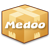version: 2.1.10

### sum

Calculate the total value of the column.

sum(\$table, \$column, \$where)
• ##### table [string]

The table name.

• ##### column [string]

The target column will be calculated.

• ##### where (optional) [array]

The WHERE clause to filter records.

sum(\$table, \$join, \$column, \$where)
• ##### table [string]

The table name.

• ##### join [array]

Table relativity for tables.

• ##### column [string]

The target column will be calculated.

• ##### where (optional) [array]

The WHERE clause to filter records.

Return: [string] The total number of the column.
```\$total = \$database->sum("account", "money");

echo "We have \$" . \$total;```